# Univariate and Multivariate Linear Regression

• Author:
• Updated date:

If we wonder to know the shoe size of a person of a certain height, obviously we can't give a clear and unique answer on this question. Nevertheless, although the link between height and shoe size is not a functional one, our intuition tells us that there is a connection between these two variables, and our reasoned guess probably wouldn’t be too far away of the true.

In case of relationship between blood pressure and age, for example; an analogous rule worth: the bigger value of one variable the greater value of another one, where the association could be described as linear. It is worth to mention that blood pressure among the persons of the same age can be understood as a random variable with a certain probability distribution (observations show that it tends to the normal distribution).

Both of these examples can very well be represented by a simple linear regression model, considering the mentioned characteristic of the relationships. There are numerous similar systems which can be modelled on the same way. The main task of regression analysis is to develop a model representing the matter of a survey as best as possible, and the first step in this process is to find a suitable mathematical form for the model. One of the most commonly used frames is just simple linear regression model, which is reasonable choice always when there is a linear relationship between two variables and modelled variable is assumed to be normally distributed.Fig. 1. Searching for a pattern. Linear regression is based on the ordinary list squares technique, which is one possible approach to the statistical analysis.

## Simple linear regression

Let (x1,y1), (x2,y2),…,(xn,yn) is a given data set, representing pairs of certain variables; where x denotes independent (explanatory) variable whereas y is independent variable – which values we want to estimate by a model. Conceptually the simplest regression model is that one which describes relationship of two variable assuming linear association. In other words, then holds relation (1) - see Figure 2, where Y is an estimation of dependent variable y, x is independent variable and a, as well as b, are coefficients of the linear function. Naturally, values of a and b should be determined on such a way that provide estimation Y as close to y as possible. More precisely, this means that the sum of the residuals (residual is the difference between Yi and yi, i=1,…,n) should be minimized:

This approach at finding a model best fitting the real data is called ordinary list squares method (OLS). From the previous expression it follows

which leads to the system of 2 equations with 2 unknown

Finally, solving this system we obtain needed expressions for the coefficient b (analogue for a, but it is more practical to determine it using pair of independent and dependent variable means)

Note that in such a model the sum of residuals if always 0. Also, the regression line passes through the sample mean (which is obvious from above expression).

Once having a regression function determined, we are curious to know haw reliable a model is. Generally, the regression model determines Yi (understand as estimation of yi) for an input xi. Thus, it worth relation (2) - see Figure 2, where ε is a residual (the difference between Yi and yi). It follows that first information about model accuracy is just the residual sum of squares (RSS):

Scroll to Continue

But to take firmer insight into accuracy of a model we need some relative instead of absolute measure. Dividing RSS by the number of observation n, leads to the definition of the standard error of the regression σ:

The total sum of squares (denoted TSS) is sum of differences between values of dependent variable y and its mean:

The total sum of squares can be anatomized on two parts; it is consisted by

1. so-called explained sum of squares (ESS) – which presents the deviation of estimation Y from the mean of the observed data, and
2. residual sum of squares.

Translating this into algebraic form, we obtain the expression

often called the equation of variance analysis. In an ideal case the regression function will give values perfectly matched with values of independent variable (functional relationship), i.e. in that case ESS=TSS. In any other case we deal with some residuals and ESS don’t reach value of TSS. Thus, ratio of ESS to TSS would be a suitable indicator of model accuracy. This proportion is called the coefficient of determination and it is usually denoted by R2

R2=ESS/TSS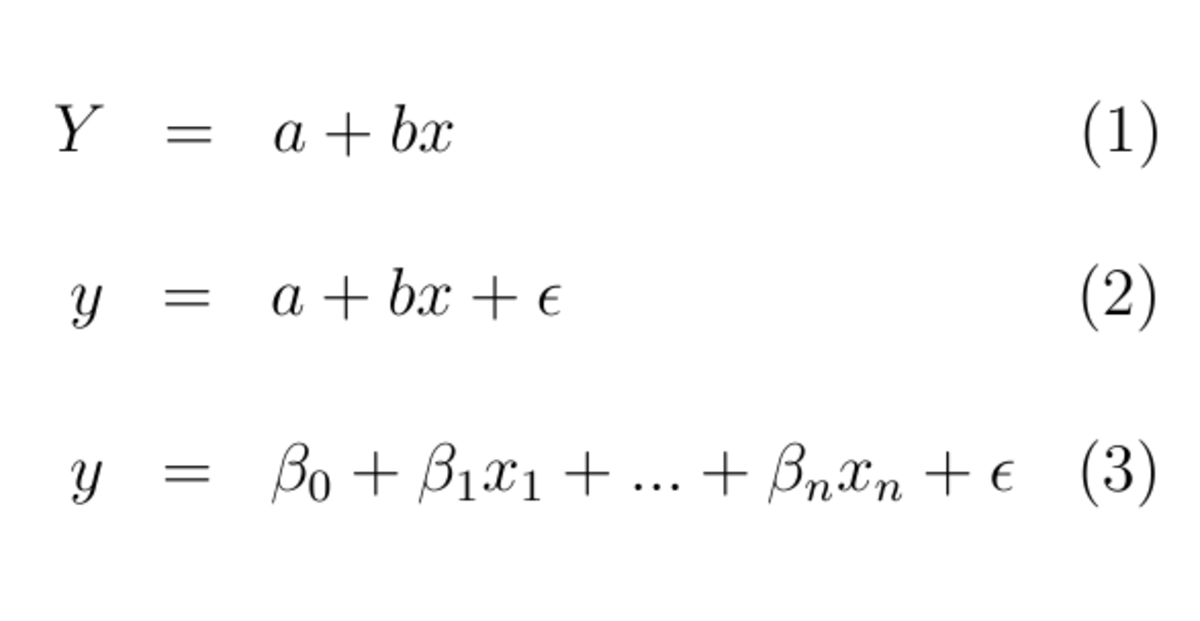Fig. 2. Basic relations for linear regression; where x denotes independent (explanatory) variable whereas y is independent variable.
 x y 165 38 170 39 175 42 180 44,5 185 43 190 45 195 46

## Case study: human height and shoe number

To illustrate the previous matter, consider the data in the next table. (Let imagine that we develop a model for shoe size (y) depending on human height (x).)

First of all, plotting the observed data (x1, y1), (x2, y2),…,(x7, y7) to a graph, we can convince ourselves that the linear function is a good candidate for a regression function.

## Regression to the mean

The term “regression” designates that the values random variable “regress” to the average. Imagine a class of students performing a test in a completely unfamiliar subject. So, the distribution of student marks will be determined by chance instead of the student knowledge, and the average score of the class will be 50%. Now, if the exam is repeated it is not expected that student who perform better in the first test will again be equally successful but will 'regress' to the average of 50%. Contrary, the student who perform badly will probably perform better i.e. will probably 'regress' to the mean.

The phenomenon was first noted by Francis Galton, in his experiment with the size of the seeds of successive generations of sweet peas. Seeds of the plants grown from the biggest seeds, again were quite big but less big than seeds of their parents. Contrary, seeds of the plants grown from the smallest seeds were less small than seeds of their parents i.e. regress to the mean of the seed size.

Putting values from the table above into already explained formulas, we obtained a=-5.07 and b=0.26, which leads to the equation of the regression straight line

Y=-5.07+0.26x

The figure below (Fig. 3) presents original values for both variables x and y as well as obtain regression line.

For the value of coefficient of determination we obtained R2=0.88 which means that 88% of a whole variance is explained by a model.

According to this the regression line seems to be quite a good fit to the data.

For the standard deviation it holds σ = 1.14, meaning that shoe sizes can deviate from the estimated values roughly up the one number of size.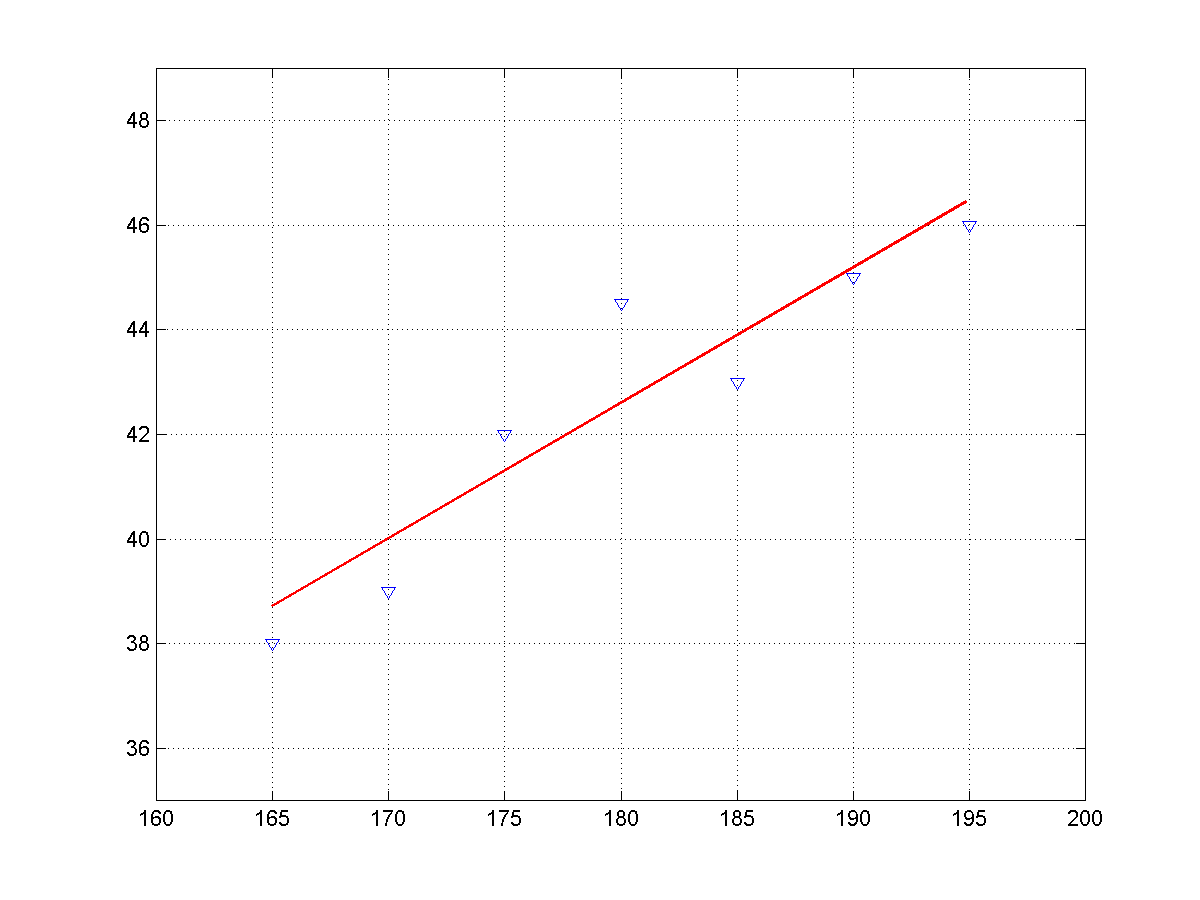Fig. 3. Comparison of the regression line and original values, within a univariate linear regression model.

## Multivariate linear regression

A natural generalization of the simple linear regression model is a situation including influence of more than one independent variable to the dependent variable, again with a linear relationship (strongly, mathematically speaking this is virtually the same model). Thus, a regression model in a form (3) - see Figure 2.

is called the multiple linear regression model. Dependent variable is denoted by y, x1, x2,…,xn are independent variables whereas β0 ,β1,…, βndenote coefficients. Although the multiple regression is analogue to the regression between two random variables, in this case development of a model is more complex. First of all, might we don’t put into model all available independent variables but among m>n candidates we will choose n variables with greatest contribution to the model accuracy. Namely, in general we aim to develop as simpler model as possible; so a variable with a small contribution we usually don’t include in a model.

## Case study: student success

Again, as in the first part of the article that is devoted to the simple regression, we prepared a case study to illustrate the matter. Let suppose that success of a student depend on IQ, “level” of emotional intelligence and pace of reading (which is expressed by the number of words in minute, let say). Let we have data presented in Table 2 on disposition.

It is necessary to determine which of the available variables to be predictive, i.e. participate in the model, and then determine the corresponding coefficients in order to obtain associated relation (3).

Table 2. Components of the student success

53

120

89

129

46

118

51

121

91

134

143

131

49

102

59

92

61

98

133

119

83

130

100

119

45

92

31

84

63

94

90

119

90

135

142

134

## Correlation matrix

The first step in the selection of predictor variables (independent variables) is the preparation of the correlation matrix. The correlation matrix gives a good picture of the relationship among the variables. It is clear, firstly, which variables the most correlate to the dependent variable. Generally, it is interesting to see which two variables are the most correlated, the variable the most correlated with everyone else and possibly to notice clusters of variables that strongly correlate to one another. In this third case, only one of the variables will be selected for the predictive variable.

When the correlation matrix is prepared, we can initially form instance of equation (3) with only one independent variable – those one that best correlates with the criterion variable (independent variable). After that, another variable (with the next biggest value of correlation coefficient) is added into the expression. This process continues until the model reliability increases or when the improvement becomes negligible.

Table 3. Correlation matrix

student success

1

IQ

0.73

1

emot.intel.

0.83

0.55

1

0.70

0.71

0.79

1

 data model 53 65.05 46 49.98 91 88.56 49 53.36 61 69.36 83 74.70 45 40.42 63 51.74 90 87.79

The next table presents the correlation matrix for the discussed example. It follows that here student success depends mostly on “level” of emotional intelligence (r=0.83), then on IQ (r=0.73) and finally on the speed of reading (r=0.70). Therefore, this will be the order of adding the variables in model. Finally, when all three variables are accepted for the model, we obtained the next regression equation

Y=6.15+0.53x1+0.35x2-0.31x3 (4)

where Y denotes estimation of student success, x1 “level” of emotional intelligence, x2 IQ and x3 speed of reading.

For the standard error of the regression we obtained σ=9.77 whereas for the coefficient of determination holds R2=0.82. The next table shows comparioson of the original values of student success and the related estimation calculated by obtained model (relation 4). Figure 4 presents this comparison is a graphical form (read colour for regression values, blue colour for original values).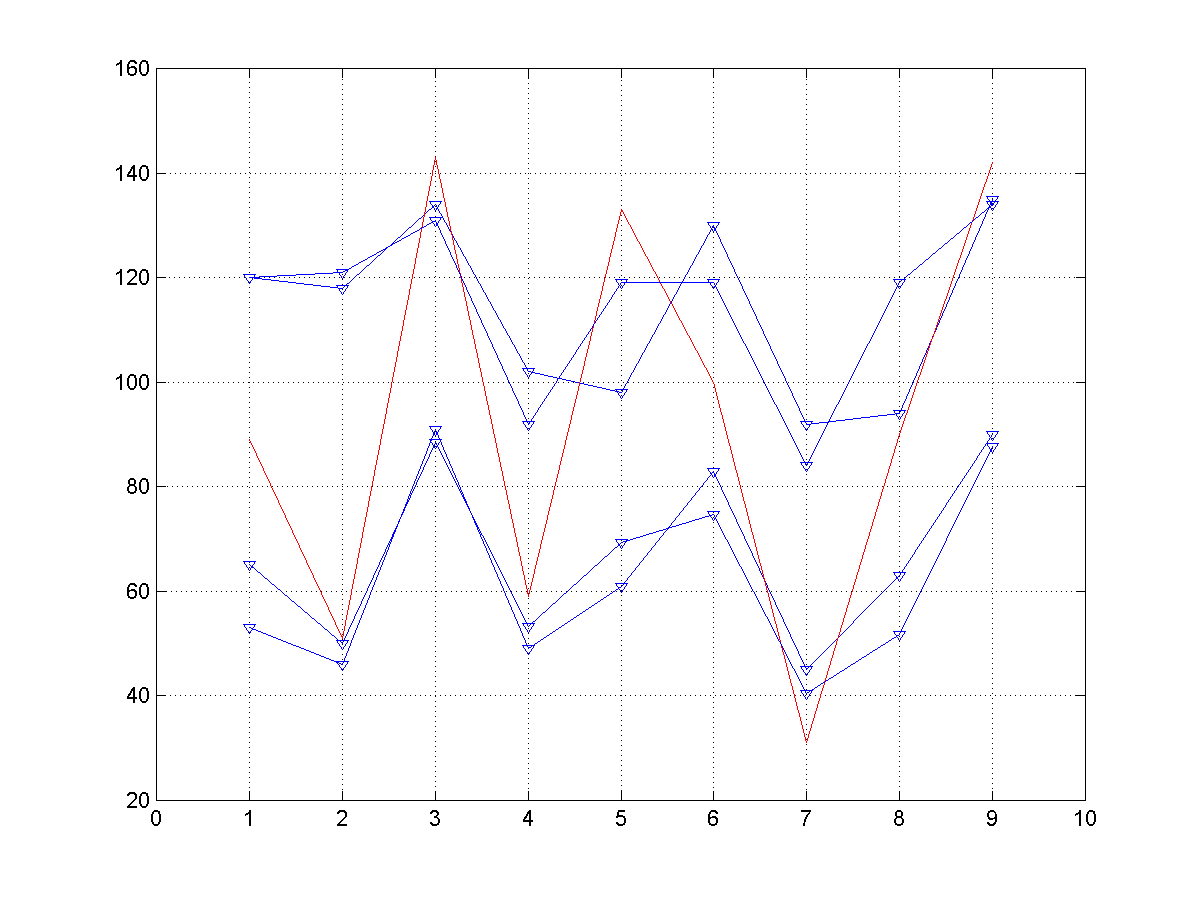Fig. 4. The regression model for a student success - case study of the multivariate regression.

## Regression analysis with software

While data in our case studies can be analysed manually for problems with slightly more data we need a software. Figure 5 shows the solution of our first case study in the R software environment. Firstly, we input vectors x and y, and than use “lm” command to calculate coefficients a and b in equation (2). Then with the command “summary” results are printed. Coefficients a and b are named “Intercept and “x”, respectively.

R is quite powerful software under the General Public Licence, often used as a statistical tool. There are many other software that support regression analysis. Video below shows how to perform a liner regression with Excel.

The Figure 6 shows solution of the second case study with the R software environment. Contrary to the previous case where data were input directly, here we present input from a file. The content of the file should be exactly the same as the content of 'tableStudSucc' variable – as is visible on the figure.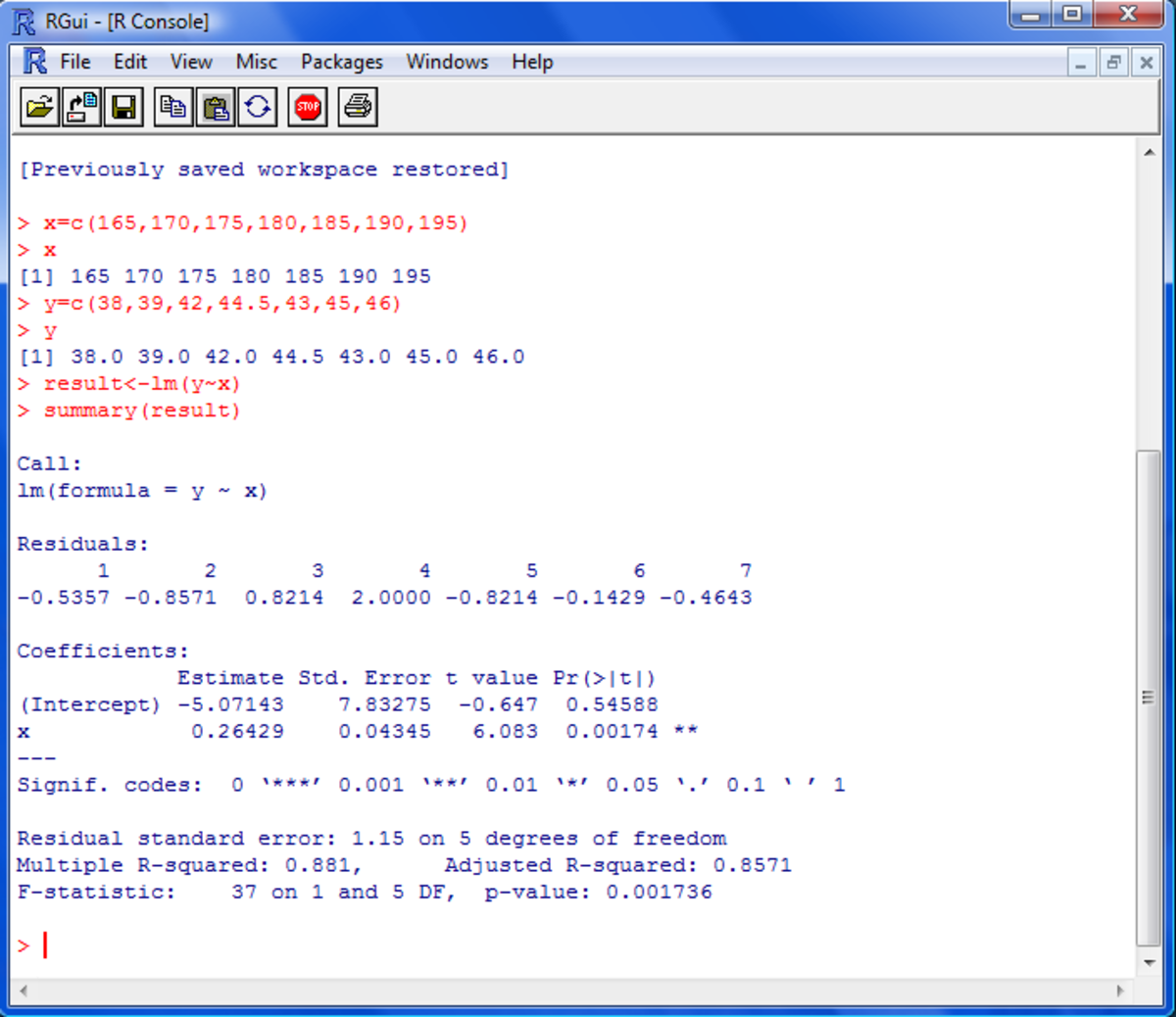Fig. 5. Solution of the first case study with the R software environment.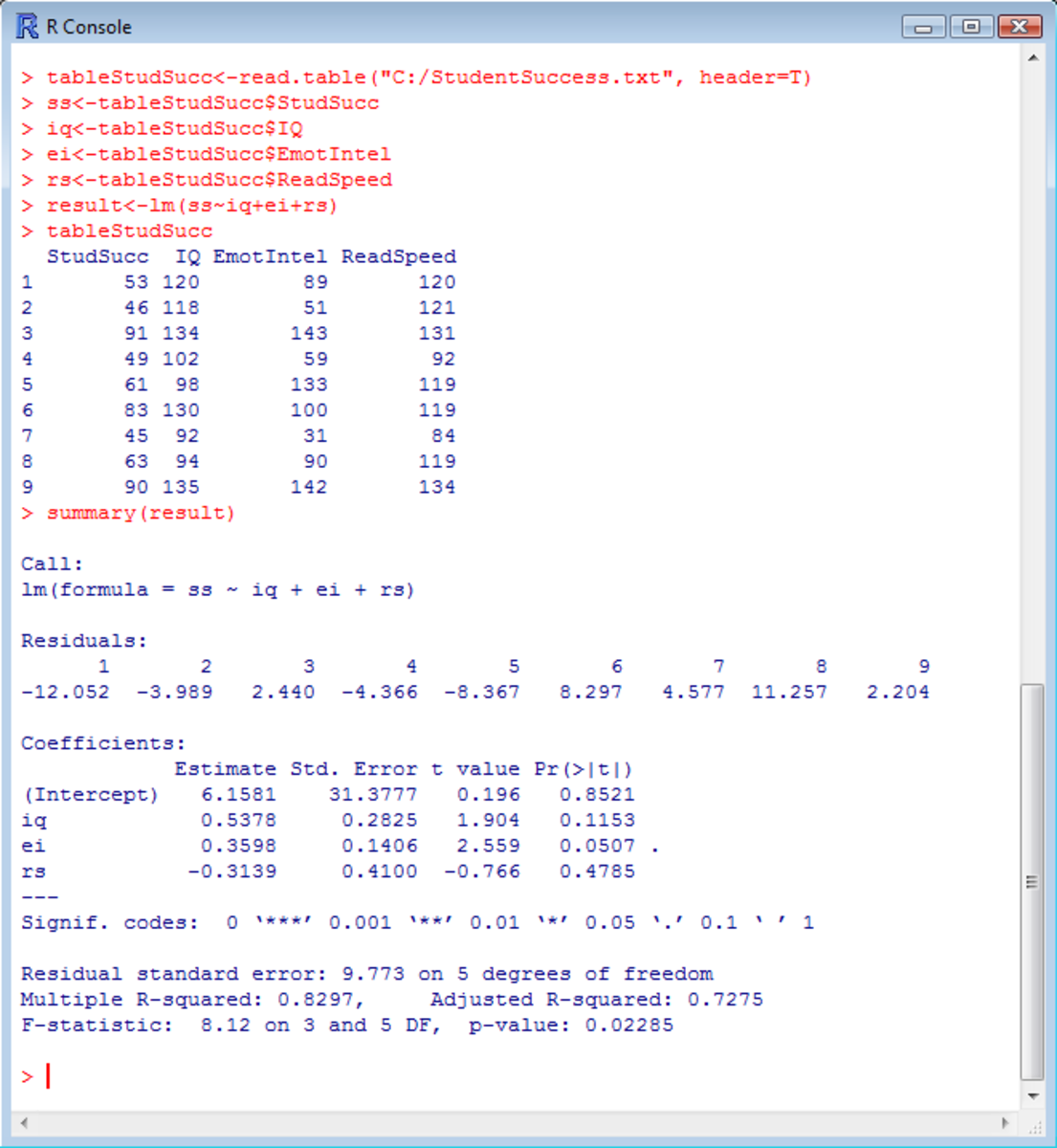Fig. 6. Solution of the second case study with the R software environment.

rajasri pateru on February 11, 2020:

Nhan Nguyen on September 27, 2017:

So is it "Multivariate Linear Regression" or "Multiple Linear Regression"?

David on January 15, 2017:

"When the correlation matrix is prepared, we can initially form instance of equation (3) with only one independent variable – those one that best correlates with the criterion variable (independent variable)"

Shouldn't the criterion variable be the dependant variable opposed to being the independant variable stated her?

Other then that, thank you very much for the clear presentation

Jose Arturo Mora Soto from Mexico on February 13, 2016:

There is a "typo" in the first paragraph of the "Simple Linear Regression" explanation, you said "y is independent variable" however "y" in a "dependent" variable.

Great explanation :-)

Peter Flom from New York on July 08, 2014:

How do you get the formulas in?

flysky (author) from Zagreb, Croatia on May 25, 2011:

Hi Horlah,

Thank you for a question. Yes, it can be little bit confusing since these two concepts have some subtle differences. So, correlation gives us information of relationship between two variables which is quantitatively expressed by correlation coefficient. The same information we get with regression concept as well, but in different form. In first case the information is presented within one figure whereas with regression we have an equation - with features that correlation coefficient between variable x and calculated values Y is the same as between x and y; and that correlation coefficient is equal to the square root of coefficient of determination (these can be easily checked in some spreadsheet – on the above data, for example…). In addition, with regression we have something more – we can to assess the accuracy with which the regression eq. can predict values (t-test is one of the basic tests on reliability of the model …) Neither correlation nor regression analysis tells us anything about cause and effect between the variables. I hope I was helpful...

Horlah from Oyo, Oyo, Nigeria on May 23, 2011:

Please help with the concept of correlation and regression or are they the same with univariate linear regression analysis?

munirahmadmughal from Lahore, Pakistan. on December 03, 2010:

"Univariate linear regression".

This is an informative hub.

It proves that human beings when use the faculties with whch they are endowed by the Creator they can close to the reality in all fields of life and all fields of environment and even their Creator.

Precision and accurate determination becomes possible by search and research of various formulas.

This in fact is a great service to humanity in what wever field it may be. Human feet are of many and multiple sizes. There is resemblance and yet individuality which is a great food for thought and scope for further research and glob-wise research. The morals of God reflect in human beings. It is the constant struggle and hardwork that opens many vistas of new and fresh knowledge. No doubt the knowledge instills by Crerators kindness on mankind. It is also His love for mankind that a few put their efforts for the sake of many and many put their efforts for the sake of few. The mutual love and affaction is causing onward march of humanity. Main thing is to maintain the dignity of mankind. It comes by respecting the rights of others honestly and sincerely. Science is in searchof truth and the ultimate truth is the Creaor Himself. Labour of all kind brings its reward and a labour in the service of mankind is much more rewardful.

May God bless all.

munirahmadmughal from Lahore, Pakistan. on December 03, 2010:

"Univariate linear regression".

This is an informative hub.

It proves that human beings when use the faculties with whch they are endowed by the Creator they can close to the reality in all fields of life and all fields of environment and even their Creator.

Precision and accurate determination becomes possible by search and research of various formulas.

This in fact is a great service to humanity in what wever field it may be. Human feet are of many and multiple sizes. There is resemblance and yet individuality which is a great food for thought and scope for further research and glob-wise research. The morals of God reflect in human beings. It is the constant struggle and hardwork that opens many vistas of new and fresh knowledge. No doubt the knowledge instills by Crerators kindness on mankind. It is also His love for mankind that a few put their efforts for the sake of many and many put their efforts for the sake of few. The mutual love and affaction is causing onward march of humanity. Main thing is to maintain the dignity of mankind. It comes by respecting the rights of others honestly and sincerely. Science is in searchof truth and the ultimate truth is the Creaor Himself. Labour of all kind brings its reward and a labour in the service of mankind is much more rewardful.

May God bless all.